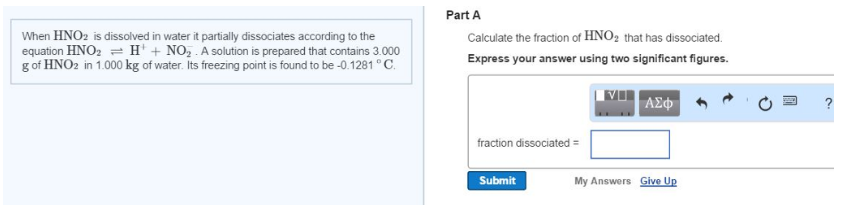# Problem: When HNO2 is dissolved in water it partially dissociates according to the equation HNO2 ⇌ H+ + NO2-. A solution is prepared that contains 3.000 g of HNO2 in 1.000 kg of water. Its freezing point is found to be -0.1281°C Calculate the fraction of HNO2 that has dissociated. Express your answer using two significant figures.

###### FREE Expert Solution
91% (262 ratings)###### Problem Details

When HNO2 is dissolved in water it partially dissociates according to the equation HNO2 ⇌ H+ + NO2-. A solution is prepared that contains 3.000 g of HNO2 in 1.000 kg of water. Its freezing point is found to be -0.1281°C

Calculate the fraction of HNO2 that has dissociated. Express your answer using two significant figures.